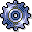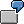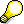Output Side VariancesUse

The output side consists of all the credits for a cost center or business process that are due to activity allocations. On the output side, the system compares the allocated actual costs with the target costs or plan costs. The output side variance categories include:

Features

Output Price Variance

An output price variance occurs if you use a price that differs from the plan price, which is calculated iteratively each month, based on plan activity. The target credit posting (= plan price x actual activity) varies from the actual credit posting (= allocation price x actual activity) on the affected cost center or business process. Output price variance can be due to several causes:

· If you use average prices instead of period-based prices

· If the capacity of the activity type is used as the basis of the price calculation.

· If you set a price manually

Output price variances are determined by the following formula:

Output price variance = actual activity x (plan price - actual price)You plan 100 hours of an activity on a cost center/activity type with plan costs of 1000 USD. The plan price is 10 USD/hr. In the actual, you use a manually-set price of 12 USD/hr. Actual activity of 110 hours produces an actual credit posting of 1320 USD (= 110 hours X 12 USD/hour) on the cost center. The target credit, however, equals 1100 USD (110 hours X 10 USD/hour). The output price variance is therefore 220 USD.If the given activity type of a cost center or a business process participates in the predistribution of fixed costs, the fixed target credit is not dependent on the operating rate, but corresponds to the plan fixed costs (see: Fixed Cost Distribution).If you have manually entered the actual quantity for the given activity type of the cost center or business process, the system calculates the output price variance corresponding to the input price variance.

Output price variance =

Target credit x (manual actual qty/output qty) - allocated Act. costs

Fixed output price variance =

Fixed target credit x (manual actual qty/output qty) - allocated Act. costs

Output Quantity Variance

The output quantity variance is the difference between the actual credits and the target credits, which is the difference between the manually-entered actual costs and the allocated actual quantities.

Variance calculation determines output quantity variance by cost element on a period basis.

Output quantity variance is based on the formula

Output quantity variance = (actual qty - manual actual qty) x plan price

Fixed output quantity variance = (actual qty – manual actual qty) x fixed plan priceVariances arising from both price and quantity differences appear as output price variances.If the corresponding activity is part of predistribution of fixed costs, the fixed target credit is not proportional to the operating rate (see: Predistribution of Fixed Costs).

Fixed Cost Variance

Fixed cost variances occur when the actual operating rate varies from the plan operating rate and some of the planned fixed costs are either underabsorbed or overabsorbed due to the credit postings.

The fixed cost variance is displayed by cost element and calculated periodically.

The fixed cost variance comprises:

• The volume variance
• The secondary fixed cost variance

The fixed cost variance is based on the following formula:

Fixed cost variance =
Plan fixed costs X (1 - operating rate) + fixed target costs - plan fixed costsThe system only determines the fixed cost variance if the operating rate varies from 100%.

Volume Variance

Volume variance shows for each cost element the over/underabsorption of fixed plan costs for each activity type or business process. It shows whether the fixed costs are covered by the actual activity. The cause of this variance category is always an actual activity varying from the plan activity – not, for example, a manually-set fixed allocation price. The results for the covering fixed costs are also affected by a manually-set allocation price. However, the cause of the variance is not the activity, but rather the structuring of the allocation price.

Volume variance is based on the formula

Volume variance = fixed plan costs x (1 – operating rate)The following data is given:

 Fixed planned costs 1,000 USD Plan activity 100 hours Calculated plan price 10/hr Actual activity 200 hours

Based on actual activity of 200 hours, you allocate fixed costs of \$2,000 (= \$10/hour X 200 hours).Because the fixed plan costs equal only \$1,000, the volume variance equals – \$1000 (= \$1,000 – \$2,000), being the overestimated portion of fixed costs due to actual activity.

If an activity type or a business process take part in the predistribution of fixed costs, the volume variance is not calculated (see: Predistribution of Fixed Costs).

Secondary Fixed Cost Variance

Secondary fixed cost variance is the difference between fixed plan costs and fixed target costs. It occurs only if a cost center or a business process carries out an activity-dependent activity input. Part of the fixed target costs (= fixed price x variable target quantity) is dependent on the operating rate. The result is a difference between the planned fixed costs and the target fixed costs.If an activity type or a business process take part in the predistribution of fixed costs, the secondary fixed cost variance is not calculated. In this case, the target fixed costs correspond to the plan fixed costs (see: Predistribution of Fixed Costs).

Remaining Variance

Remaining variance indicates a difference between target costs and allocated actual costs that cannot be assigned to any of the following variance categories:

• Output price variance
• Output quantity variance
• Fixed cost variance

This can be due to the following causes:

• You deactivated calculation of variance categories on the output side.
• You deactivated all variance categories. If this is the case, the sum of all variances determined is displayed in the remaining variance.

The system does not calculate and display the remaining variance by cost element, but does so for the entire Controlling object.

The following special cases are also possible:

• Minor differences appear as remaining variance if you wish.
• If no target costs exist for the Controlling object, this also results in all variances appearing under remaining variance.

Idle Capacity Cost Variance

The system cannot determine idle capacity cost variance directly. However, you can also calculate it from the volume variance and the fixed portion of the output price variance if the following prerequisites are fulfilled:

• The system calculated period-based prices for the activity on the basis of capacity.
• No subsequent adjustment occurs in the actual.

In this case, the idle capacity cost variance is calculated as follows:

Idle capacity cost variance = volume variance + fixed output price variance

To display the idle capacity cost variance, you have two options:

• Variance calculation determines the volume variance and the output price variance

You can then use a report to display the two variance categories together and identify them as idle capacity cost variance.

• Idle capacity cost variance appears as the sole variance category on the output side

If this is the case, you must deactivate all variance categories on the output side with the exception of remaining variance. The idle capacity cost variance then appears to the remaining variance.

However, you must define the variance variant for the entire controlling area.

Output Side Variance Categories